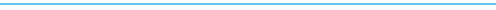Count, Add, Subtract! Fun with Math, Music, and Movement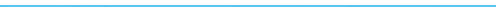Words and Music by Hap Palmer ©Hap-Pal Music / www.happalmer.com
Introduction
Who knew math could be such fun! A colorful variety of musical styles make basic addition and subtraction facts easy to learn. These songs strike a balance between problem solving and memorization with an emphasis on developing mathematical thinking skills.

The songs are organized sequentially in a progression of skills and problem solving strategies with the understanding that children have individual patterns and rates of internalizing these concepts.

1. Count Up, Count Down – Counting from 0 to 10, and 10 to 0
2. Naming Numbers – Counting from 0 to 20, and 20 to 0
3. One More, One Less – Adding and subtracting 1
4. Doubles Facts – Learning doubles facts
5. Add One To The Double – Using the doubles plus one strategy
6. Switcheroo – Understanding the commutative property
7. Skip Count – Skip counting by 2s, 5s, and 10s
8. Two More, Two Less – Adding and subtracting 2
9. Ways To Get To Ten – Learning combinations that equal 10
10. Ten Plus – Adding single digit numbers to 10
11. Make Nine Into Ten – Using ten plus facts to solve nine plus facts
12. All Aboard To Add And Subtract – Understanding the relationship between
13. Counting By Twos With The Odd Numbers – Skip counting with odd numbers
14. The Five Seven Twelve Family – Recognizing the 5, 7, 12 fact family and it's       relation to the doubles fact 6 + 6
15. Five Ate Thirteen – A fun way to memorize the facts in the 5, 8, 13 family

These songs are useful for a wide range of ages from pre-school to third grade. Start at the level of the students with whom you are working. For example, if a child already knows how to count from 0 to 20 and from 20 back down to 0, you can skip the first two songs and move to the doubles facts. When doubles facts are mastered, move to the concept of doubles plus one. Every song has an instrumental or no answers version to challenge the students and test their knowledge.

Each song focuses on a mathematical skill or problem solving strategy. Emphasize that there is more that one strategy to solve any problem. The goal is that the child can provide a quick response and also explain or verify that the answer is correct. With this approach, students can come up with answers as fast as rote memorization and the facts stay with them longer. In fact, after employing a strategy many times, children often memorize the fact and no longer use the strategy. Having a way to figure out the basic facts, will help students memorize them (Rathmell).

Many students suffer from math anxiety (Beilock and Ramirez). Music and Movement can reduce math anxiety. Getting the body in motion stimulates deeper breathing, which is an effective anecdote to anxiety. Many movement activities are included in this guide.

Studies on the relative difficulty of 100 single digit addition facts show the following:
1. Most children find doubles facts the easiest to remember.
2. Larger sums are generally more difficult than smaller sums.
3. The ten most difficult non-zero, single digit addition facts are:
7 + 9, 8 + 9, 7 + 8, 7 + 5, 5 + 7
9 + 7, 9 + 6, 5 + 8, 8 + 6, 7 + 6
Interestingly, this research was first done in 1939 (Murray) and was confirmed in 1993 (Frobisher et al.) suggesting that little has changed to influence the relative difficulty of addition facts.

Many of the songs in this collection suggest strategies that will help students answer the ten most difficult facts.

7 + 9 - Switcheroo, Make Nine Into Ten
8 + 9 - Switcheroo, Make Nine Into Ten, Add One To The Double
7 + 8 - Doubles Facts, Add One To The Double
7 + 5 - The Five Seven Twelve Family
5 + 7 - The Five Seven Twelve Family
9 + 7 - Ten Plus, Make Nine Into Ten
9 + 6 - Ten Plus, Make Nine Into Ten
5 + 8 - Five Ate Thirteen
8 + 6 - Doubles Facts, Skip Count, Two More Two Less
7 + 6 - Doubles Facts, Add One To The Double

Initially, some children may find zero confusing when it is the first addend in a fact.
Often 4 + 0 is easier to answer than 0 + 4. Understanding the commutative principle (featured in the song Switcheroo) shows children that 0 + 4 can be viewed as 4 + 0.

Currently, the more difficult facts are often referred to as "no strategy" or "left over facts." These are facts that don't fit the strategies of 1 more, 2 more, doubles, or doubles plus 1. Included in this list are:
3 + 5, 3 + 7, 4 + 6, 4 + 7, 5 + 7, 5 + 8 and 6 + 3.

Here are songs that suggest strategies for solving these problems:
3 + 5 Doubles Facts, Two More Two Less
3 + 7 Ways To Get To Ten
4 + 6 Ways To Get To Ten
4 + 7 Ways To Get To Ten, Ten Plus
5 + 7 The Five Seven Twelve Family
5 + 8 Five Ate Thirteen
6 + 3 All Aboard To Add And Subtract

Initially, some children may find zero confusing when it is the first addend in a fact,
for example, 0 + 4. Often 4 + 0 is easier to answer. Understanding the commutative principle (featured in the song Switcheroo) shows children that 0 + 4 can be viewed
as 4 + 0.

The 100 basic addition facts are shown in the following chart:

 0 1 2 3 4 5 6 7 8 9 0 0 1 2 3 4 5 6 7 8 9 1 1 2 3 4 5 6 7 8 9 10 2 2 3 4 5 6 7 8 9 10 11 3 3 4 5 6 7 8 9 10 11 12 4 4 5 6 7 8 9 10 11 12 13 5 5 6 7 8 9 10 11 12 13 14 6 6 7 8 9 10 11 12 13 14 15 7 7 8 9 10 11 12 13 14 15 16 8 8 9 10 11 12 13 14 15 16 17 9 9 10 11 12 13 14 15 16 17 18

By learning key strategies students can quickly answer most of these facts through reason and logic while developing a deeper understanding of mathematics. The strategies also help students memorize the basic single digit math facts. Studies show that students who have the basic facts memorized do better in college readiness exams (Ansari, Price et al.) (Sparks)

Lyrics and Activities:
These songs are designed for both group and individualized learning. They are effective in a variety of situations from one child in the home or car to a school classroom setting. Encourage children to sing along and call out the answers.

It is helpful to add a visual component to the songs. Children learn better when they can both see and hear the information. A simple number line written on the board and a pointer is all you need for most of the songs. Point to and tap the numbers as they are named in the song. As children become familiar with the concepts and skills, they enjoy playing the role of the teacher and pointing to the numbers.

You can also include movement activities and add kinesthetic sensory input. A variety of activities are suggested from finger and hand motions to full body vigorous motions that promote fitness and reduce math anxiety.

Many of the suggested activities use number cards. These are easily made with permanent marker pen and cardboard. Plastic circular tops from a variety of food packaging also work well. They are very durable and easy to find. When you are working with large groups of children you can use "imaginary" number cards and have children visualize the number cards as they move.

In this teaching guide, two or three possible activities are given for each song. These activities are suggestions. There are many more variations and possibilities awaiting your creative impulses.

Words and Music: Hap Palmer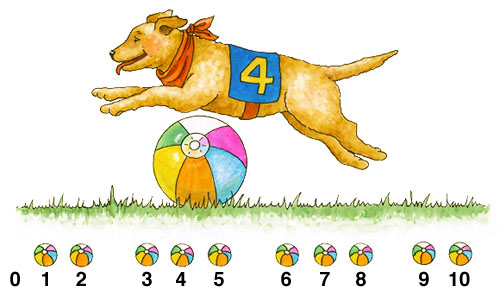Goals:
• Identify numbers and count from 0 to 10.
• Count from 0 to 10 and back down from 10 to 0.
• Understand the concept of "nothing" and recognizing '0' (zero) as its symbol.
• Count to tell the number of objects up to 10.

Lyric:
We're doin' a count up
We're doin' a count up
We're doin' a count up from zero
We know what we say
And we say what we know
And we know we can count up from zero
0, 1, 2,   3, 4, 5,    6, 7, 8,    9, 10, Yay!

We're doin' a count down
We're doin' a count down
We're doin' a count down to zero
We know what we say
And we say what we know
And we know we can count down to zero
10, 9, 8,    7, 6, 5,    4, 3, 2,    1, 0, Yay!

Repeat All

We count up, count down
Up to 10 and back again
Count up, count down!

Activities:
1. Write the numerals 0 through 10 on the board in a horizontal line from left to right. Encourage children to sing along as you point and tap the numbers. As children learn the numbers, let them point and tap.

2. Draw 10 large dots on the board or place 10 objects on a felt board (ducks, stars, balls, etc.) Number each object 1 through 10. Place the numeral '0' to the left with no object. Tap and count the 10 objects as each number is recited in the song. This activity reinforces the concept that zero represents nothing and helps children see the correspondence of numbers to concrete objects. Research shows that preschoolers' ability to count actual objects in order relates to higher math scores in first grade. (Manfra)

Movement Activities:
Count Up, Count Down Finger Dance – Suggested cues:
1. Let your fingers dance with the music as you sing about counting up.
2. To count up, start with your fist for '0' and count up until 10 fingers are showing.
3. When you reach 10, wiggle and wave your 10 fingers and shout "Yay!"
5. Countdown by bringing down fingers one at a time until no fingers are showing.
6. Wave your fist to show zero and shout, "Yay!"

Bean Bag Toss to Ten – Place the number cards 0 through 10 clockwise in a circle on the floor. Put a bean bag on each number card 1 through 10 as the children count the bags. Place a container (basket, box etc.) in the center of the circle. Each child in the class stands behind a number. When they hear their number they try to throw the bean bag into the container. When counting backwards the children retrieve the bags one by one. Each time "zero" is heard, no bean bag is thrown, reinforcing the idea that zero means nothing.

Note: When children throw bean bags into the container some land inside the container and some may land outside the container. When you get to 10 you can stop the song and ask, "How many bags do you see outside the container? How many do you guess are inside the container?" This provides an opportunity to encourage mathematical thinking. Feel free to use the pause button on your playback device whenever a teachable moment arises.

Run, Jump, and Count – Place a hurdle in the middle of an open area. The hurdle can be made with 2 empty milk containers and a mailing tube or broomstick as a cross bar. Ten people line up on one side of the hurdle. On the other side of the hurdle, place the number cards 0 through 10 side by side. Number the players 1 through 10. The players listen for their number then run and jump over the hurdle and line up behind their number card. During the count down they jump the hurdle again and return to their original places in line. Students see 10 people counted and see that no one stands by 0 which shows that zero is the same as nothing.1. Use the instrumental version of this song (#16) to count higher sequences. For example, you can count from 95 to 105 in the following way:
We're doin' a count up, we're doin' a count up
We're doin' a count up from 95
We know what we say and we say what we know
And we know we can count up from 95
95, 96, 97 … 98, 99, 100 … 101,102,103 … 104, 105, Yay!

Note we are using the same pattern as the basic version; 3 groups of 3 numbers
followed by a group of 2 numbers and cheering Yay!

2. Place the number 0 on the left side of the board and 10 on the right side of the board so there is a large space between. Have children estimate where the number '5' would go. Do the same with other numbers in the sequence. This will help children develop a "Mental Number Line" which helps them develop a better memory for numbers (Thompson, Siegler). You can do the same activity with the numbers 0 through 20. Ask, "where would 10 go? ... 5? ... 15? This is a fun way to set up a number line for the next song "Naming Numbers."

Words and Music: Hap Palmer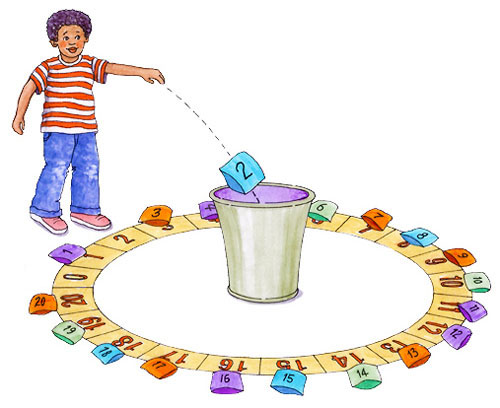Goals:
• Identify numbers and count from 0 to 20.
• Count forwards from 0 to 20 and backwards from 20 to 0.
• Understand the concept of "nothing" and recognizing '0' (zero) as its symbol.
• Find the missing number when the music pauses.
• Count to tell the number of objects up to 20.

Lyric:
Counting forwards, counting backwards,
Naming numbers as we go
Counting forwards, counting backwards,
Naming numbers as we go
0, 1, 2, 3, 4, 5, 6, 7, 8, 9, 10, 11, 12, 13, 14, 15, 16, 17, 18, 19, 20

Now we turn around and we head back down
'Til we make it all the way to zero
20, 19, 18, 17, 16, 15, 14, 13, 12, 11, 10, 9, 8, 7, 6, 5, 4, 3, 2, 1, 0

Counting forwards, counting backwards,
Naming numbers as we go

What's missing? I wonder.
Can you find the missing number?
0, 1, 2, __ 4, 5, 6, __ 8, 9, 10, __
12, 13, __ 15, 16, __ 18, 19, __

Now we turn around and we head back down
'Til we make it all the way to zero
20, 19, __ 17, 16, __ 14, 13, __
11, 10, 9, __ 7, 6, 5, __ 3, 2, 1, __

Counting forwards, counting backwards,
Naming numbers as we goActivities:
1. Write the numerals 0 through 20 on the board. Tap each number as it is named in the song. Children can see the progression moving forward as they count from zero to twenty and backward as they count from twenty to zero.

2. Draw 20 large dots on the board or place 20 objects on a felt board (ducks, stars, circles, etc.) Let the number zero go by, then tap and count the 20 objects as each number is recited in the song. This activity reinforces the concept that zero is nothing and helps children see the correspondence of numbers to concrete objects.

Movement Activities:
Count and Stand – Each child holds a number card 1 through 20 and starts in a seated position. Counting forward children stand when their number is called. Counting backward they sit when their number is called. When the music pauses, the child holding the missing number waves the card in the air. Note that no one stands or sits with the number 0 is named. This shows that 0 means nothing.

Run and Count – For the aerobic version of the above activity, each child holds a number card 1 through 20 and starts in a standing position. Children run in place for a count of 20 and hold their card up when their number is called. They start running when they hear "zero" and stop when they hear, "twenty." Next, they take a short rest and then run in place and count backwards from twenty to zero. Counting while moving helps develop automatic counting skills and reduces math anxiety. (Beilock and Ramirez.) Each time the music stops the children stop moving and call out the missing number. Note that no one holds up a card when the number 0 is named. This shows that 0 means nothing.

Bean Bag Toss to Twenty – Lay the number cards 0 through 20 clockwise in a large circle on the floor. Put a bean bag on each number card 1 through 20 as the children count the bags. Do not put a bean bag on the zero card. Place a basket in the center of the circle. Each child in the class stands behind a number. When they hear their number they try to throw the bean bag into the basket. When counting backwards the children retrieve the bags one by one. Each time "zero" is heard, no bean bag is thrown, reinforcing the idea that zero means nothing. In the verses that follow, whenever the music stops, everyone calls out the missing number.

1. Using the instrumental version (#17) try other counting sequences. For example, you could count forward from 30 to 50 then backwards from 50 to 30. Finding the missing number would go as follows:

What's missing? I wonder. Can you find the missing number?
30, 31, 32, __ 34, 35, 36, __ 38, 39, 40, __
42, 43, __ 45, 46, __ 48, 49, __

Now we turn around and we head back down
'Til we make it all the way to zero
50, 49, __ 47, 46, __ 44, 43, __
41, 40, 39, __ 37, 36, 35, __ 33, 32, 31, __

2. Play the game "Lucky Number." Make a spinner with the numbers 0 through 20 in a circle on a piece of wood or thick cardboard. Attach an arrow that spins on a nail or tack in the center of the circle of numbers. At the end of the song everyone should be holding or standing by a number card. Spin the arrow and see who has the "Lucky Number." You can also do this activity with the song "Lucky Numbers" from the CD Learning Basic Skills Through Music Volume II.

Back to Titles

Words and Music: Hap PalmerGoals:
• Understand the words "more" and "less."
• Add 1 by counting forward one.
• Recognize an upward pattern as the answers increase in value.
• Subtract 1 by counting back one.
• Recognize a descending pattern as the answers decrease in value.

Lyric:
3 + 1 is one more than 3
3 + 1 is (bell) 4
4 + 1 is one more than 4
4 + 1 is (bell) 5
5 + 1 is (bell) 6, 6 + 1 (bell) is 7
7 + 1 is (bell) 8, 8 + 1 (bell) is 9

One more, one less
If you can count you can add and subtract
Count forward to add and backwards to subtract

9 - 1 is one less than 9
9 - 1 is (bell) 8
8 - 1 is one less than 8
8 - 1 is (bell) 7
7 - 1 is (bell) 6, 6 - 1 is (bell) 5
5 - 1 is (bell) 4, 4 - 1 is (bell) 3

One more, one less
If you can count you can add and subtract
Count forward to add and backwards to subtract

Activity:
Write the numerals 3, 4, 5, 6, 7, 8 and 9 on the board. Tap the answer to each addition and subtraction fact when the bell rings. As children learn the facts, they call out the answer. They can also hold up fingers to indicate the answers.

Movement Activity:
Fox and Rabbits - Outline a large circle on the floor with chalk or a long rope.

Nine children pretend to be rabbits and one child pretends to be a fox. To start, three rabbits jump about inside the circle. Five rabbits and the fox stand outside the circle.

Each time the bell rings, one more rabbit jumps inside the circle. (The teacher may want to ring a bell to reinforce the bell sound in the recording) The first verse ends with 9 rabbits inside the circle.

In the second verse, each time the bell rings, the fox jumps inside the circle and taps one rabbit then jumps outside the circle with the rabbit. At the end of the second verse, only 3 rabbits are left inside the circle.

Optionally, this can become a game of tag where the rabbits dart about and try to be one of the 3 rabbits remaining in the circle.

1. When children have learned the adding one facts, they can call out the answers with the no answers version (#18)

2. Write each addition and subtraction fact on a small card. Put the cards in a box and shake them up. Draw the cards out one by one and have the students answer the facts in a random order.

Words and Music: Hap PalmerGoals:
• Memorize the single digit doubles facts.
• Sing the doubles facts in order.
• Recognize a pattern of how the sums increase in steps of two.

Lyric:
Doubles facts are fun to say
Chant them as you sway
Hear that rockin' rhythm
That they have within them
1+1 is 2, 2 +2 is 4, 3+3 is 6, 4+4 is 8, 5+5 is 10
6+6 is 12, 7+7 is 14, 8+8 is 16, 9+9 is 18

When you put these facts in order
A pattern comes to view
You'll see the sums of doubles facts
Increase in steps of two
1+1 is 2, 2+2 is 4, 3+3 is 6, 4+4 is 8, 5+5 is 10
6+6 is 12, 7+7 is 14, 8+8 is 16, 9+9 is 18

When two addends are the same
Doubles is their name
See how many facts you know
That's our doubles game
1+1 is _ , 2+2 is _ , 3+3 is _ , 4+4 is _ , 5+5 is _
6+6 is _ , 7+7 is _ , 8+8 is _ , 9+9 is _

2, 4 ,6, 8, What do we appreciate?
Doubles, doubles, Yay, doubles!Activity:
Place the even numbers 2 through 18 on
the board.
Tap each doubles fact answer as it is named
in the song.

Movement Activities:
Doubles Partner Dance – Children move in pairs with a partner. They jump, bounce or dance in place as they sing the doubles facts. As they move, each person holds up fingers to represent one of the doubles addends. For example to show 6 + 6, each person holds up 6 fingers. Then the partners put their fingers together to make 12 and show the answer.

Doubles On The Line – Place number cards for the even numbers 2 through 18 in order, end to end, on the floor. Children work in pairs standing on either side of the number line. They hold up fingers to represent the addends as together jump to each doubles fact answer.

1. Have children call out the answers with the no answers version (#19).

2. Write each doubles fact on a small card. Put the cards in a box and shake them up. Draw the cards out one by one and have the students answer doubles facts in a random order.

Words and Music: Hap Palmer

Goals:
• Review the doubles facts.
• Learn to use the doubles plus one strategy.
• Understand how you can go from what you know to what you don't know.
• Introduce the strategy of doubles plus two. (See follow up activity.)

Lyric:
Add one to the double you know
That's the easy way to go
You'll know a whole lot more than you did before
By adding one to the double you know

2 + 2 is 4, 2 + 3 is just one more, 2 + 3 is 5
3 + 3 is 6, 3 + 4 is just one more, 3 + 4 is 7
Because 4 + 4 is 8, we know 4 + 5 is 9,
Because 5 + 5 is 10, we know 5 + 6 is 11

You don't have to use our fingers
Hesitate or linger
At an ever faster rate

2 + 2 is 4, 2 + 3 is one more, 2 + 3 is 5
3 + 3 is 6, 3 + 4 is one more, 3 + 4 is 7
4 + 4 is 8, 4 + 5 is one more, 4 + 5 is 9
5 + 5 is 10, 5 + 6 is one more, 5 + 6 is 11

Once you get them in your brain
Doubles facts will help explain
How you get from what you know
To what you do not know

6 + 6 is 12, 6 + 7 is 13
7 + 7 is 14, 7 + 8 is 15
Because 8 + 8 is 16, we know 8 + 9 is 17,
Because 9 + 9 is 18, we know 9 + 10 is 19

Add one to the double you know
That's the easy way to go
You'll know a whole lot more than you did before
By adding one to the double you know

Activity:
Place the numbers 4 through 19 on the board
4, 5, 6, 7, 8, 9, 10, 11, 12, 13, 14, 15, 16, 17, 18, 19.
Sing and tap the answer to each fact.

Movement Activity:
Lay the number cards 4 through 19 end to end in a line on the floor. The student stands by the sum of each doubles fact, then jumps forward one card to land by the sum of the doubles fact plus one. Students can also share the number line and work in pairs. One student stands on each side of the number line. As students learn the song, they can both sing along and move to the answers.1. Students call out the answers to each doubles fact and each doubles plus one fact using the no answers version (#20).

2. Try the strategy of doubles plus two. Use the same approach of starting from a doubles fact. For example, students can chant or sing:
3 + 3 is 6
3 + 5 is just two more.
3 + 5 is 8

3. Try the strategy of doubles minus one. Use the same approach of starting from a doubles fact. For example, students can chant or sing:
4 + 4 is 8
4 + 3 is just one less.
4 + 3 is 7

Words and Music: Hap Palmer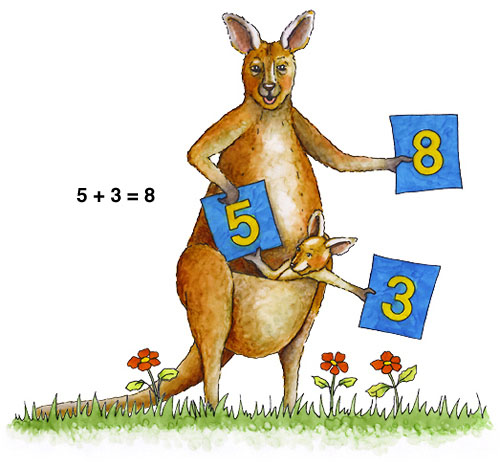Goals:
• Understand the meaning of the words "addend" and "sum."
• Recognize that when you switch the order of the addends,
the sum remains the same (commutative property.)
• Learn that when you add zero to a number, the number stays the same.
• Use fingers to show addition facts with addends from 0 to 5.
• Review basic addition facts: 2 + 3, 3 + 2, 4 + 0, 0 + 4, 1 + 4, 4 + 1,
2 + 5, 5 + 2, 4 + 5, 5 + 4, 3 + 4, 4 + 3, 4 + 2, 2 + 4, 3 + 5, 5 + 3.

Lyric:
2 + 3, 3 + 2 either way you say it the sum is 5
4 + 0, 0 + 4 either way you say it the sum is 4

Switchin' those addends is a cinch to do
You can change the order with a switcheroo

1 + 4, 4 + 1 either way you say it the sum is 5
2 + 5, 5 + 2 either way you say it the sum is 7

Switcharoo, Switcheroo,
Switchin' those addends is a cinch to do

4 + 5, 5 + 4 either way you say it the sum is 9
3 + 4, 4 + 3 either way you say it the sum is 7

Switchin' those addends is a cinch to do
You can change the order with a switcheroo

4 + 2, 2 + 4 either way you say it the sum is 6
3 + 5, 5 + 3 either way you say it the sum is 8

Switcheroo, Switcheroo,
Switchin' those addends is a cinch to do

Activities:
Finger Game - Show the first addend with the left hand and the second addend with the right hand. Cross hands to show the addends reversed.

Call and Response - Listen to the first fact, switch the addends and sing the fact in reverse then sing out the answer.

For an extra challenge, combine the above two activities!

Partners with Number Line - Place number cards 0 through 9 end to end in a line on the floor. Participants work in pairs, one person standing on each side of the number line. As the addends are called, one person stands by the first addend and the other by the second addend. When the addends are reversed they "switcheroo" and trade addends. When they hear the phrase, "Either way you say it the sum is ...", both people run and stand by the answer.Note: Earlier versions of Switcheroo use addends of 0 – 8. This newer version uses addends of 0 – 5 so children can use the fingers of each hand to show the addends and then cross hands to show addends reversed.

version (#21).

Words and Music: Hap PalmerGoals:
• Count by twos to twenty.
• Count by fives to fifty.
• Count by tens to a hundred.
• Clap in sets of two, five and ten.

Lyric:
Skip count in steps of two
Count by twos to twenty
You'll get to twenty twice as quickly
When you count by twos
2, 4, 6, 8, 10, 12, 14, 16, 18, 20
Super speed! 2, 4, 6, 8, 10, 12, 14, 16, 18, 20
Instrumental (clap in sets of 2)

Skip count in steps of five
Count by fives to fifty
You'll get to fifty oh so quickly
When you count by fives
5, 10, 15, 20, 25, 30, 35, 40, 45, 50
Super speed! 5, 10, 15, 20, 25, 30, 35, 40, 45, 50
Instrumental (clap in sets of 5)

Skip count in steps of ten
Count by tens to a hundred
In ten steps you'll reach a hundred
When you count by tens
0, 20, 30, 40, 50, 60, 70, 80, 90, 100
Super speed! 10, 20, 30, 40, 50, 60, 70, 80, 90, 100
Instrumental (clap in sets of 10)

Activity:
Students count by twos to twenty. In the instrumental interlude that follows, they clap in sets of two. In the second verse they count by fives to fifty and clap in sets of five. In the third verse they count by tens to a hundred and clap in sets of ten.

Movement Activity:
Participants do the following sequence as they sing, chant and count:

Skip count in steps of two count by twos to twenty
Take 8 marching steps (or 8 hop steps) in place.

You'll get to twenty twice as quickly when you count by twos
Slowly bounce and squat for 7 counts, moving lower with each count.

2, 4, 6, 8, 10, 12, 14, 16, 18, 20
Start in a low squatting position and slowly stand. Finish on your tiptoes, and reach high when you get to 20. Here is an example of how it might look:
2 – Squat very low.
4 – Come up a few inches.
6 – Come up a few more inches.
8 – Stand with knees bent.
10 – Stand with knees straight.
12 – Lift arms halfway up.
14 – Lift arms up a little more.
16 – Reach up with straight arms.
18 – Rise up on toes.
20 – Stand on tiptoes reaching and stretching as high as possible.

Super speed! 2, 4, 6, 8, 10, 12, 14, 16, 18, 20
Jump in rhythm with the music and count by 2s to 20.

Instrumental (step and clap in sets of 2)

Repeat the above sequence skip counting in 5s and 10s. When the sequence is learned, participants can step and jump while moving in a circle and do this activity in the style of a folk dance.

Use the instrumental version (#22) to try other counting patterns. For example, you could count by threes to thirty or by fours to forty.

Skip count in steps of three
Count by threes to thirty
You'll get to thirty oh so quickly
When you count by threes
3, 6, 9, 12, 15, 18, 21, 24, 27, 30
Super speed! 3, 6, 9, 12, 15, 18, 21, 24, 27, 30
Instrumental (clap in sets of 3)

Skip count in steps of four
Count by fours to forty
You'll get to forty very shortly
When you count by fours
4, 8, 12, 16, 20, 24, 28, 32, 36, 40
Super speed! 4, 8, 12, 16, 20, 24, 28, 32, 36, 40
Instrumental (clap in sets of 4)

Words and Music: Hap Palmer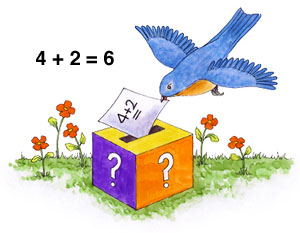Goals:
• Understand the words "more" and "less."
• Add 2 by counting forward 2 from the larger number.
• Subtract 2 by counting back 2.

Lyric:
3 + 2 is 2 more than 3
3 + 2 is (bell) 5
4 + 2 is 2 more than 4
4 + 2 is (bell) 6
5 + 2 is (bell) 7, 6 + 2 (bell) is 8
7 + 2 is (bell) 9, 8 + 2 (bell) is 10

Two more, two less
If you can count you can add and subtract
Count forward to add and backwards to subtract

10 - 2 is 2 less than 10
10 - 2 is (bell) 8
9 - 2 is 2 less than 9
9 - 2 is (bell) 7
8 - 2 is (bell) 6, 7 - 2 is (bell) 5
6 - 2 is (bell) 4, 5 - 2 is (bell) 3

Two more, two less
If you can count you can add and subtract
Count forward to add and backwards to subtract

Activity:Write the numerals 3, 4, 5, 6, 7, 8, 9, and 10 on the board. Tap the number line two times in rhythm with the music after each addition problem. Move up (or back) one step with each tap to arrive at the answer. Have the children call out each answer.

Movement Activity:
The children stand in an open area facing the numbers on the board. The teacher repeats the activity described above. As the numbers are tapped, the children take two small jumps forward then call out the answer. For example, after "3 plus 2 is _" the children take two small jumps forward and call out the answer, "5". To subtract, the children take two jumps backward and call out the answer.

1. Have children call out the answers with the no answers version (#23).

2. Write each addition and subtraction fact on a small card. Put the cards in a box and shake them up. Draw the cards out one by one and ask the students to answer the facts in a random order.

Back to Titles

Words and Music: Hap Palmer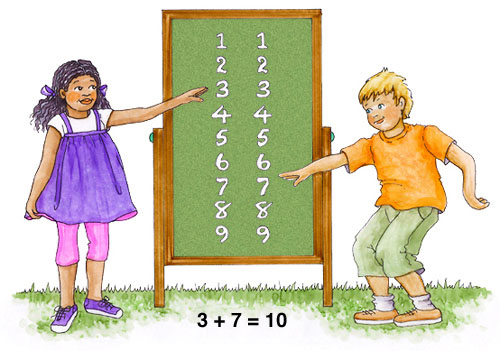Goals:
• Learn single digit addition combinations that equal ten:
1 + 9, 2 + 8, 3 + 7, 4 + 6, 5 + 5, 6 + 4, 7 + 3, 8 + 2, 9 + 1
• Use rhyming words to help in memorizing these combinations.
• Recognize a pattern of how the first addend increases in steps of
one as the second addend decreases in steps of one.
• Mix the combinations up in a random order. (See follow up activity.)

Lyric:
Let's find some ways we can get to ten
Again and again

1 + 9 works just fine
2 + 8 there's no debate
3 + 7 has always been
One more way we can get to 10

4 + 6 fits the mix
5 + 5 and we'll arrive
6 + 4 we all adore
'Cause it's one more way we can get to 10

7 + 3 works perfectly
8 + 2 will surely do
9 + 1 gets it done
And it's one more way we can get to 10

1 + (Bell) __ works just fine
2 + (Bell) __ there's no debate
3 + (Bell) __ has always been
One more way we can get to 10

4 + (Bell) __ fits the mix
5 + (Bell) __ and we'll arrive
6 + (Bell) __ we all adore
'Cause it's one more way we can get to 10

7 + (Bell) __ works perfectly
8 + (Bell) __ will surely do
9 + (Bell) __ gets it done
And it's one more way we can get to 10
And we can get to 10 again and againActivity:
Place two vertical columns of numerals
from 1 through 9 on the board.

Point and tap each combination of
numbers as they are named in the song. Viewers will see the pattern of how you move down the first column and up the second column.

Children work in pairs and tap the numbers. One child taps the first addend in the left column, and the other taps the second addend in the right column.

Movement Activities:
Grouping Objects (Deconstructing Ten)

Materials: 10 objects, (buttons, container tops, bean bags, etc.) and two hoops.

The participants work with a partner. The partners sit side by side with 2 hoops (optional) in front.

Place 10 objects in the hoop in front of person on the right.

Ask, "Can you and your partner find a way to group the objects so you can quickly see there are 10 without having to count each item?" (Example: 2 groups of 5. Have children describe their arrangement)

The players pass objects from R to L until there are 10 on the L. (Try this activity first without the music. Recite the following lyric)

1 + 9 works just fine
2 + 8 there's no debate
3 + 7 has always been
One more way we can get to 10

4 + 6 fits the mix
5 + 5 and we'll arrive
6 + 4 we all adore
'Cause it's one more way we can get to 10

7 + 3 works perfectly
8 + 2 will surely do
9 + 1 gets it done
And it's one more way we can get to 10

Ask, "Can you think of another addition combination that equals ten?" (10 + 0)

At the end of the third verse, with the words, "And it's one more way we can get to ten" the tenth and final object is passed. Now all 10 objects are on the left.

The participants quickly move to the other side of the hoops and face the opposite direction so that the 10 objects are on the R and do the activity again.

Explain, "this time there will be a slight delay before you hear the answer. See if you can say the answer in the pause."

Variation: This activity can also be done individually

Partners on the Line – Place the number cards 1 through 9 end to end in a line on the floor. Children work in pairs one person standing on each side of the number line. The child on the left stands by the first addend while the child on the right stands by the second addend of each ten combination. Children will see the pattern of how they move in opposite directions along the number line. They start far apart, come closer, pass side by side, and end far apart. After the song you can ask, "What ten combination brings you closest to your partner? Which ten combinations take you the farthest apart?" Questions for discussion engage the learners more fully.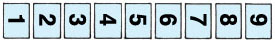Meet in the Middle – For larger groups of children (18 participants), place two parallel columns of number cards 1 through 9 on the floor. Have one child stand by each number card. As each pair of numbers is called, the two children meet in the middle, circle each other and return to their places.

Bean Bag Toss – Use hula-hoops or ropes to make two circles 3 to 5 feet apart on the floor. Place 10 bean bags in one of the circles. One player stands in each circle. Each time the bell rings, the player in the circle with the bean bags picks up a bag and tosses it to the person in the other circle who catches the bag and places it inside his/her circle.
At the end of the third verse, the words, "And it's one more way we can get to ten"
are the signal to throw the tenth and final bean bag. After a short instrumental section, the song is repeated and the players reverse roles. This activity shows all the combinations that make 10 in a concrete way.

1. Have students name the missing addend that will make a sum of ten using the no answers version (#24).

2. Include the concept of nothing or zero in combinations that make 10. Ask students:
• What would you add to 10 to make 10?
• What would you add to 0 to make 10?

3. Write the numerals 0 through 10 each on a separate small card. Put the cards in a box and shake them up. Draw the cards out in a random order and have students name the missing addend that makes a sum of ten.

Back to Titles

Words and Music: Hap Palmer

Goals:
• Learn the ten plus facts and solve problems that have 10 as an addend.
• Interpret quantities from 11 to 19 as a group of 10, plus another group.
(Example: 17 would be a group of 10 and a group of 7.)

Lyric: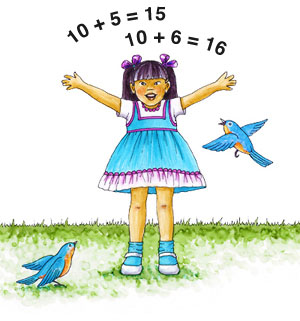Ten plus is just a must
A powerful tool that you can trust
Ten plus can be your friend
For adding sums of more than 10

10 + 1 is 11, 10 + 2 is 12
10 + 3 is 13, 10 + 4 is 14
10 + 5 is 15, 10 + 6 is 16

Repeat Verse 1

10 + 7 is 17, 10 + 8 is 18
10 + 9 is 19, 10 + 10 is 20

Repeat Verse 1

Now let's review in super speed
From ten plus one to ten plus ten

10 + 1 is 11, 10 + 2 is 12
10 + 3 is 13, 10 + 4 is 14
10 + 5 is 15, 10 + 6 is 16
10 + 7 is 17, 10 + 8 is 18
10 + 9 is 19, 10 + 10 is 20

Activity:
Write the numerals 10 through 20 on the board. Point to each answer as it is named in the
song. Have students hold up fingers to represent the number that is being added to
10 and call out the answer.

Movement Activity:
Ten Plus Agility Run – Place the number cards 10 through 20, end to end, in a line on the floor. With larger groups, players can share each number line and work together in pairs. One person stands on either side of the number line starting by the number 10. As each fact is named, they run to the sum. They quickly return to the ten card and repeat the sequence for each fact. The distance traveled will be farther for each fact creating a fun challenge that develops math skills, agility, and physical fitness. When the facts are chanted "super speed" players can just step to each answer without running back to
10 each time.1. Have children call out the answers using the no answer version (#25)

2. Write the numerals 11 through 20 on the board. Point to each number in a random order and ask students, "What number could you add to 10 to make this sum?"

Back to Titles

Words and Music: Hap Palmer

Goals:
• Show how 10+ facts can help answer 9+ facts.
• Learn the addition facts 9 + 5, 9 + 6, 9 + 7, and 9 + 8.
• Show how you can use facts you know to answer facts you don't know.

Lyric:
To add 9 + 5, think 10 + 5
10 + 5 is 15
Now all you need to do is subtract 1
9 + 5 is 14

To add 9 + 6, think 10 + 6
10 + 6 is 16
Now all you need do is subtract 1
9 + 6 is 15

Chorus:
You make the 9 into 10
And adding is easy to do
Just add the other number and take away one
And the answer is there for you

To add 9 + 7, think 10 + 7
10 + 7 is 17
Now all you need do is subtract 1
9 + 7 is 16

To add 9 + 8, think 10 + 8
10 + 8 is 18
Now all you need do is subtract 1
9 + 8 is 17

Repeat Chorus

You make 9 into 10
You make 9 into 10Activity:
Place the numbers 14, 15, 16, 17, and 18 on the board. These are the sums of all the addition facts included in this song. Tap each answer as it is named in the song. Children can see the pattern of subtracting one from the total of the 10+ sum to arrive at the 9+ sum. For example, if 10 + 5 = 15, subtracting 1 from 15 gives the answer to 9 + 5 … 9 + 5 = 14.

Movement Activity:

Place the number cards 14 through 18, end to end, on the floor. Children run to the sum of the 10+ fact then jump back one card to show the sum of the 9+ fact. During the chorus, children hold up 9 fingers, then 10 fingers, and dance around the numbers.

1. Have children call out the answers using the no answers version (#26)

2. Find other 9+ facts with which you can use the "Make Nine Into Ten" strategy. Examples: 9 + 2, 9 + 3, 9 + 11, 9 + 12, 90 + 40, 90 + 50, etc.

Back to Titles

12. All Aboard To Add and Subtract
Words and Music: Hap PalmerGoals:
• Understand the relationship between addition and subtraction.
• Recognize the number bond showing that 9 is the whole and 6 and 3 are the parts.
• Recognize the number bond showing that 12 is the whole and 8 and 4 are the parts.
• Learn the addition and subtraction facts in the 3, 6, 9 family, and the 4, 8, 12 family.

Lyric:
Let's take a trip that travels on a triangle track
We'll take it to the top, turn around and come back
Toot, toot, all aboard, to add and subtract
Let's take the 3, 6, 9 line, here we go!
3 + 6 is 9, 9 - 6 is 3,
3 + 6 is 9, 9 - 6 is 3
Go the other way!
6 + 3 is 9, 9 - 3 is 6
6 + 3 is 9, 9 - 3 is 6

Let's take a trip that travels on a triangle track
We'll take it to the top, turn around and come back
Let's take the 4, 8, 12 line, here we go!
4 + 8 is 12, 12 - 8 is 4
4 + 8 is 12, 12 - 8 is 4
Go the other way!
8 + 4 is 12, 12 - 4 is 8
8 + 4 is 12, 12 - 4 is 8

Just take a little trip on a triangle track
Toot, toot, all aboard, to add and subtract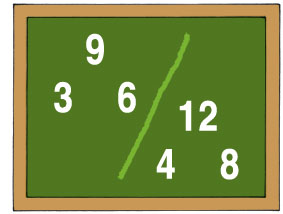Activity:
Place the numerals 3, 6, 9 and 4, 8, 12 on the board in triangles. Point to each number as it is named in the song.

Movement Activity:
Repeat the above activity with students standing in an open area facing the board.

Each person imagines they are standing behind the numbers 3, 6, and 9 placed in a small triangle just like they see on the board.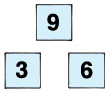As the numbers are tapped on the board, players jump in a triangle shape on the floor. In the second verse of the song, repeat the activity using the numbers 4, 8, and 12.

Note: If the group is small, you can provide three number cards for each player.
On one side of the cards write the numerals 3, 6, and 9. On the other side write 4, 8, and 12. Place the number cards 3, 6, and 9 out on the floor in a triangle. The player starts at the number 3 and runs to each card as it is named in the song. When the funny voice says, "Go the other way" the player starts at the number 6 and moves in the opposite direction to each number card. Halfway through the song, flip the numbers over to 4, 8, and 12 and repeat the activity with the 4, 8, 12 fact family.

1. Play the above games using the no answers version (#27). Students call out the answers to each addition and subtraction problem.

2. Sing this song using other combinations such as the 3, 5, 8 family.Let's take a trip that travels on a triangle track
We'll take it to the top, turn around and come back
Let's take the 3, 5, 8 line, here we go!
3 + 5 is 8, 8 – 5 is 3
3 + 5 is 8, 8 – 5 is 3
Go the other way!
5 + 3 is 8, 8 – 3 is 5
5 + 3 is 8, 8 – 3 is 5

Back to Titles

13. Countin' By Twos With Odd Numbers
Words and Music: Hap PalmerGoals:
• Understand the meaning of "even" and "odd" numbers.
• Skip count by twos with odd numbers from 1 to 23.
• Recognize how this ability makes adding and subtracting 2 easier.

Lyric:
1, 3, 5, 7, 9
Ladybugs hidin' in a pumpkin vine
11, 13, 15, 17
Jelly beans jumpin' on a trampoline
19, 21, 23
Katydids singin' in a sycamore tree

We're countin' by twos with the odd numbers
Skipping right over the even numbers
Startin' at one, havin' some fun
Countin' by twos with the odd numbers

1, 3, 5, 7, 9
Ladybugs hidin' in a pumpkin vine
11, 13, 15, 17
Jelly beans jumpin' on a trampoline
19, 21, 23
Katydids singin' in a sycamore tree

1, 3, 5, 7, 9, 11, 13, 15, 17, 19, 21, 23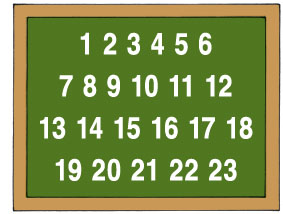We're countin' by twos with the odd numbers!

Activity:
Write the numerals 1 through 23 on the board.
Tap the odd numbers and skip over the even numbers.

Movement Activity:
Place the number cards for the odd numbers 1 through 23 end to end in a line on the floor.Participants start on either side of the number line standing by the 1 card and receive
the following instructions:
• Start with three quick jumps landing by 1, 3, and 5
• Jump on the beat landing by 7 and 9.
• Dance in place and chant, "Ladybugs hidin' in a pumpkin vine."
• Jump and land by 11, 13, 15, and 17.
• Dance and chant, "Jelly beans jumpin' on a trampoline."
• Jump and land by 19, 21, and 23.
• Dance and chant, "Katydids singin' in a sycamore tree."
Dance back to the start of the number line and repeat the sequence. Finish by running back to the start and jumping from 1 to 23 in rhythm without stopping to chant.

Write out the words on a poster board or chalkboard. Have children read and chant this rap with the instrumental version (#28)

Back to Titles

14. The Five, Seven, Twelve Family
Words and Music: Hap Palmer

Goals:
• Show how the fact 5 + 7 is related to the doubles fact 6 + 6.
• Recognize the number bond showing that 12 is the whole, and 5 and 7 are the parts.
• See how 5, 7, and 12 show two addition facts and two subtraction facts.
(5 + 7 = 12, 7 + 5 = 12, 12 - 5 = 7, 12 - 7 = 5).

Lyric:
6 + 6 is 12
Everyone knows it's true
6 + 6 is 12
Now see what we can do

Take 1 from a 6
And give it to the other 6
Heave ho and away we go
Take 1 from a 6
And give it to the other 6
Now we have 5 and 7
And just like 6 + 6, 5 + 7 is 12!
5 + 7 is 12, 12 - 7 is 5
7 + 5 is 12, 12 - 5 is 7

Will be one of these three: 5, 7, 12
That's our happy family 5, 7, 12
5 + 7 is (bell)...12
12 - 7 is (bell)...5
7 + 5 is (bell)...12
12 - 5 is (bell)...7
5, 7, 12, that's our happy family 5, 7, 12!

Activity:
On a chalk or felt board place two sets of 6 objects.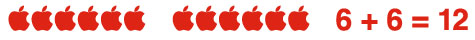Point to each group of 6 with the words "6 + 6 is 12." Next, erase or move one object and add it to the other group of 6.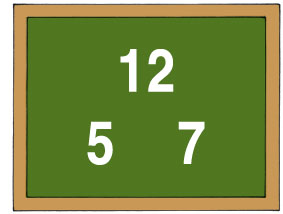Go to the numbers 5, 7, and 12 in a triangle shape and tap each number as it is named in the song.

Movement Activity:
Repeat the above activity with students standing in an open area facing the board.

Each person imagines they are standing behind the numbers 5, 7, and 12 placed in a small triangle just like they see on the board.As the numbers are tapped on the board, players jump in a triangle shape on the floor.

2. Find other facts that allow you to take one fromthereby creating an easy to solve doubles fact.
Example: 5 + 3 has the same sum as 4 + 4.

3. Look at the numbers shown on the cover of this
CD (5, 3, 8). Name all the addition and subtraction
facts you can think of that use these numbers.

Back to Titles

15. Five Ate Thirteen
Words and Music: Hap PalmerGoals:
• Recognize the number bond where 13 is the whole, and 5 and 8 are the parts.
• Learn a fun way to memorize the facts in the 5, 8, 13 fact family. (These facts can
be more challenging because they are not easily solved with the strategies of one
more, two more, doubles, or doubles plus one.)
• Understand the words addend and subtrahend.
• Review the commutative principle by seeing that changing the order of
the addends does not change the sum.

Lyric:
Chorus:
Five ate thirteen tangerines
Five ate thirteen fresh sardines
Whenever you see 5 + 8
Think: Five ate thirteen

When you hear the problem
5 + 8 is (bell)... 13
8 + 5 is (bell)... 13

Repeat Chorus

You can do subtraction
13 - 5 is (bell)... 8
Make the 8 the subtrahend
13 - 8 is (bell)... 5

Repeat Chorus

Whenever you see 5 + 8
Think: Five ate thirteen, yum, yum,
Five ate thirteen!Activity:
Place the numerals 5, 8, and 13 in a triangle on the board. Tap each number as it is named. Students sing along with the chorus and call out the answer to each problem when the bell rings.

Movement Activity:
Write the numbers 5, 8, and 13 on the board in a triangle shape as in the above activity. Each student imagines the numbers 5, 8, and 13 in a small triangle on the floor. Students jump in a triangle shape as they watch the numbers being tapped on the board. (If the group is not large you can use sets of number cards 5, 8, and 13 for each person. Students jump and land near each number as it is named in the song.)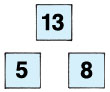Five ate thirteen tangerines
Jump 3 times landing by 5, 8, and 13.

Five ate thirteen fresh sardines
Jump 3 times landing by 5, 8, and 13.

Whenever you see 5 + 8

Think: Five ate thirteen

Repeat Chorus

When you hear the problem
5 + 8 is (bell)... 13

8 + 5 is (bell)... 13

You can do subtraction
13 - 5 is (bell)... 8

Make the 8 the subtrahend
13 - 8 is (bell)... 5

Repeat Chorus

Once students learn the sequence they can add their own motions and create a
"Five Ate Thirteen Dance." Here is an example:

Five ate thirteen tangerines
Jump to 5, 8, and 13 then clap 3 times while wiggling hips.

Five ate thirteen fresh sardines
Jump to 5, 8, and 13 then clap 3 times while wiggling hips.

Whenever you see 5 + 8

Think: Five ate thirteen

Glossary:
Absolute Number - The distance between a number and zero. Absolute numbers can
be positive or negative.

Example: In 2 + 3 = 5, the 2 and the 3 are addends.

Addition - Joining two or more numbers or quantities.

Basic Addition Facts - A basic addition fact is defined as the sum of two one-digit

to solve. You adjust the numbers being added or subtracted to make the problem
easier to do mentally.

Composite Number - A number that can be divided evenly
by numbers other than 1 or itself.

Commutative - The condition that a group of quantities connected by operators gives the same result whatever the order of the quantities involved, e.g., a + b = b + a.

Commutative Property -In mathematics, a binary operation is commutative if changing the order of the operands does not change the result. The commutative property of addition states that the order of the addends can be changed and the sum will remain the same.

Count - To recite or list numbers in order.

Count Down - To recite numerals in descending order, as during a countdown.

Count Up - To count all the things or people in a group.

Counting On - A strategy for finding the number of objects in a group without
counting every member of the group. For example, if a stack of books
is known to have 8 books and 3 more books are added to the top, it is not
necessary to count the stack all over again. One can find the total by counting
on—pointing to the top book and saying "eight," following this with "nine, ten,
eleven. There are eleven books now."

Difference - The answer to a subtraction problem.

Estimate - To find a number that is close to the exact answer. A close guess of the
actual value, usually with some thought or calculation involved.

Equation - A statement showing the equality of two expressions usually separated by
left and right signs and joined by an equals sign.

Even Number - Any whole number (integer) that can be divided exactly by 2. The
last digit will be 0, 2, 4, 6 or 8. In other words, a whole number that is a multiple of 2
and that has 0, 2, 4, 6 or 8 in the ones place.

Fact Family - A fact family (also called number bonds) shows the relationship between a number and the parts that combine to make it. A whole thing is made up of parts. If you know the parts, you can put them together (add) to find the whole. If you know the whole and one of the parts, you can take away the part you know (subtract) to find the other part. Fact families show the inverse relationship between addition and subtraction.

Integer - A whole number; a number that is not a fraction. An integer can be
positive, negative or 0.

Less Than - When one number is of smaller or lesser value than another number.
The symbol for this concept is "<".

Minuend - The quantity from which another quantity, the subtrahend, is to be
subtracted. In the equation 9 - 6 = 3, the minuend is 9.

More Than, Greater Than – When a number is of higher or larger value than another
number. The symbol for this concept is ">".

Negative Number - A number that is less than zero.
.
Number - A number is an abstract concept while a numeral is a symbol used to
express that number. "Three," "3″ and "III" are all symbols used to express the same
number (or the concept of "threeness").

Number Bonds - Number bonds (or fact families) are pictorial representations of part-part-whole number relationships.  They consist of a minimum of 3 circles that are connected by lines.    The "whole" is written in the first circle and its "parts" are written in the adjoining circles.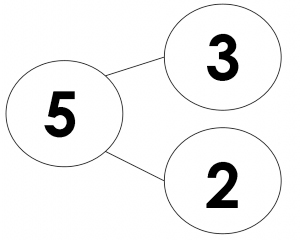For example, this number bond shows that 5, can be split into two
parts, 3 and 2. Number bonds help to build a deeper understanding of math facts.

Numeral - A written representation of a number or fixed numerical quantity; a figure,
symbol, or group of these denoting a number.

Odd Number - Any whole number (integer) that cannot be divided exactly by 2. The
last digit will be 1, 3, 5, 7 or 9. In other words, a whole number that is not a multiple
of 2 and that has 1, 3, 5, 7, and 9 in the ones place.

Operand - The quantity on which an operation is to be done.
In mathematics, an operand is the object of a mathematical operation, a quantity on
which an operation is performed.

Operation - An addition, subtraction, multiplication, or division number sentence - a
way to show a relationship between numbers.

Patterns - Recognizable regularities in situations such as in nature, shapes, events,
sets of numbers. For example, spirals on a pineapple, snowflakes, geometric designs
on quilts or wallpaper, the number sequence {0, 4, 8, 12, 16,...}.

Positive Number - A number that is greater than zero.

Prime Number - A number that can be divided evenly only by 1 or itself. It must be
a whole number greater than 1. Examples: 2, 3, 5, 7, 11, 13, 17, 19, 23, 29, 31, 37,
41, 43, 47, 53 59.

Subtraction - Taking one or more numbers (quantities) away from another number.

Subtrahend - A quantity or number to be subtracted from another. In the equation
13 - 8 = 5, the subtrahend is 8.

Sum - The result of adding two or more numbers.
Example: 9 is the sum of 2, 4 and 3.

Zero Property – The zero addition/subtraction property says a number does not change when adding or subtracting zero from that number. Examples: 4 + 0 = 4, 5 – 0 = 5. The multiplication property says that zero times any number is equal to zero. Examples: 3 x 0 = 0, 0 x 8 = 0.

References:
Ansari, Daniel and Gavin Price et al. "Why Mental Arithmetic Counts: Brain Activation During Single-Digit Arithmetic Predicts High School Math Scores," Journal of Neuroscience 2013

Beilock, Sian and Gerado Ramirez. "Math Anxiety, Working Memory, and Math Achievement in Early Elementary School," Journal of Cognition and Development 2012

Buchholz, Lisa. "Learning Strategies for Addition and Subtraction Facts: The Road to Fluency and the License to Think."  NCTM Journal, Teaching Children Mathematics March 2004.

Isaacs, Andrew and William Carroll. "Strategies for Basic-Facts Instruction." NCTM Journal, Teaching Children Mathematics May 1999.

Leutzinger, Larry. Facts That Last (Addition): A Balanced Approach to Memorization. Chicago: Creative Publications, 1999.

Leutzinger, Larry. Facts That Last (Subtraction): A Balanced Approach to Memorization. Chicago: Creative Publications, 1999.

Manfra, Louis. "Associations between Counting Ability in Preschool and Mathematic Performance in First Grade among a Sample of Ethnically Diverse, Low-Income Children", Journal of Research in Childhood Education. 2013

National Council of Teachers of Mathematics (NCTM) Principles and Standards for School Mathematics. Reston, Va: NCTM, 2000.

National Governors Association Center for Best Practices, Council of Chief State School Officers. Common Core State Standards for Mathematics. Washington D.C. 2010

Orton, Anthony and Leonard Frobisher: Insights Into Teaching Mathematics. London: Cassell, 1996.

Van de Walle, John A. Elementary and Middle School Mathematics: Teaching Developmentally. New York: Addison Wesley Longman, 2001

Rathmell, Edward C. "Using Thinking Strategies to Learn Basic Facts." Developing Computational Skills. The 1978 Yearbook of the National Council of Teachers of Mathematics, Marilyn Suydam (editor). National Council of Teachers of Mathematics, Reston, Va. 1978.

Saundry, Carole, Sharon Jeroski et al. Math Makes Sense Grade 1. Toronto, Ontario, Canada: Pearson Education 2007

Sparks, Sarah D. "Little Numbers Add Up to Big Differences in High School Math Tests" Education Week/Inside School Research blog.
http://blogs.edweek.org/edweek/inside-school-research/2013/01/little
numbers add up to big d.html
. January 15, 2013.

Thompson, Clarissa A. and Robert S. Siegler. "Child's 'Mental Number Line' Affects Memory for Numbers. Psychological Science, Journal of the Association for Psychological Science. September 12, 2010

Saskatoon Public Schools. Mental Math Strategies. Online Learning Centre Resource. http://olc.spsd.sk.ca/de/math1-3/p-mentalmath.html. 2005.

Wei Sun, Wei and Joanne Zhang "Teaching Addition and Subtraction Facts: A Chinese Perspective"  NCTM Journal, Teaching Children Mathematics. September 2001.

Credits
Engineer: Tim Bryson
Arrangers: Miriam Mayer, Hap Palmer
Producer: Hap Palmer
Drums: Tom Walsh
Guitars: Fino Roverato, Larry Giannecchini, Hap Palmer
Keyboards: Robert Irving
Bass: Jim Garafalo
Pedal Steel Guitar: Doug Livingston
Accordion: Dart Zubis
Percussion: Brian Kilgore
Trumpet: Chris Tedesco
Trombone, Tuba: Toby Holmes
Saxophone, Clarinet, Flute: Glen Berger, Geoff Nudell
Background Vocals: Amy Keys, Gigi Worth, Hap Palmer
Young Peoples' Chorus: Megan Schulze, Yael Karoly, Gari Geiselman
Talia Heimanson
Chanters: Kayla Schulze, Ariana Rouhani, Gari Geiselman, Talia Heimanson,
Yael Karoly, Megan Schulze

Mastering Engineer: Ruairi O'Flaherty
Recorded at: Robert Irving Studio
Designer Illustrator: Carole Schumacher Onaitis
Guide Book Layout: Stan Onaitis
Guidebook Editor: Paula Benjamin Little

All songs written and performed by Hap Palmer

Teaching Guide for Count, Add, Subtract! by Hap Palmer

Consultants
Megan Franke
Professor and Chair
UCLA Education Department
Los Angeles, CA

Kevin North
Math Specialist
UCLA Lab School
Los Angeles, CA

Wendy Young
Elementary Math Coach
Travis Unified School District
Travis, CA

Reagan Tunstall
Startzville Elementary School
Canyon Lake, Texas

Kelly Schulze
Kindergarten Teacher
Serrania School
Woodland Hills, CA

Betsy Stane
Kindergarten Teacher
Serrania School
Woodland Hills, CA

Jane Brolsma
Springfield, OR

Linda Chapman
Roseville, CA

Vickie Prater
Agoura High School
Agoura, CA

More Math Songs from Hap Palmer
Songs that relate to counting, addition and subtraction from earlier
Hap Palmer recordings.

Numbers and Counting

Boogie Down And Cruise – CD: Can A Jumbo Jet Sing The Alphabet?

Can Cockatoos Count By Twos? – CD: Can Cockatoos Count By Twos?

Five Pennies Make A Nickel – CD: Two Little Sounds

Follow Along – CD One Little Sound

Lucky Number March – CD: Learning Basic Skills - Volume II

Numbers Can Tell About You – CD: Can Cockatoos Count By Twos?

One, Two, Buckle My Shoe – CD: Early Childhood Classics

On The Count of Five – CD: Sally The Swinging Snake

Saliendo a la ciudad (Going Out On The Town) – CD: Divirtámonos aprendiendo

Stepping Out On The Town – CD: Can A Cherry Pie Wave Goodbye?

Take Me Out To The Ball Game – CD: Can A Jumbo Jet Sing The Alphabet?

Ten Wiggle Worms – CD: So Big

Ten Wiggle Worms II – CD: So Big

The Number March – CD: Learning Basic Skills - Volume I

Thirty Second Challenge – CD: Can Cockatoos Count By Twos?

Tug-A-Tug To Twenty-Two – CD: Can A Jumbo Jet Sing The Alphabe?t

We Discover Number Patterns – CD: Two Little Sounds

Bean Bag Shake – CD: Rhythms On Parade

Do You Know – CD: Math Readiness

How Many Are Here? (Addition) – CD: One Little Sound

How Many Are Here? (Addition and Subtraction) – CD: Two Little Sounds

Jumping To Add And Subtract – CD: Can Cockatoos Count By Twos?

Tall Tremendous Tens And Wee Wonderful Ones – CD: Two Little Sounds

Tap Out The Answer – CD: Learning Basic Skills – Volume 5

Ten Monkeys In A Tree – CD: Two Little Sounds